# Chem 212 Final Review Flashcards

Set Details Share
created 3 years ago by mcmagie7
265 views
updated 3 years ago by mcmagie7
Page to share:
Embed this setcancel
COPY
code changes based on your size selection
Size:
X

1

The rising of liquids in narrow tubes against the force of gravity is called

- capillary action

- cohesion

- specific elasticity

- surface tension

- viscosity

capillary action

2

procaine hydrochloride (M = 272.8g/mol) is used as a local anesthetic. Calculate the molarity of a 2.51 m solution of procaine hydrochloride in water which has a density of 1.057 g/mL

- 1.98 M

- 1.58 M

- 1.87 M

- 1.67 M

- 1.15 M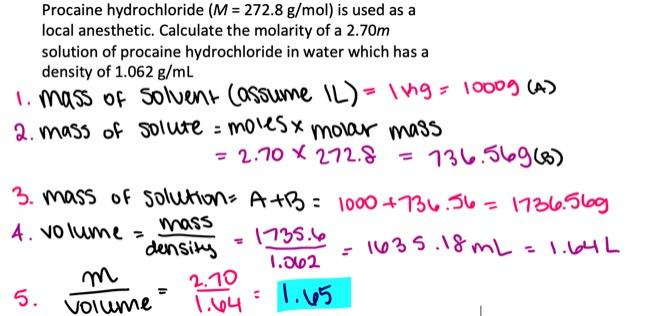1.58 M

3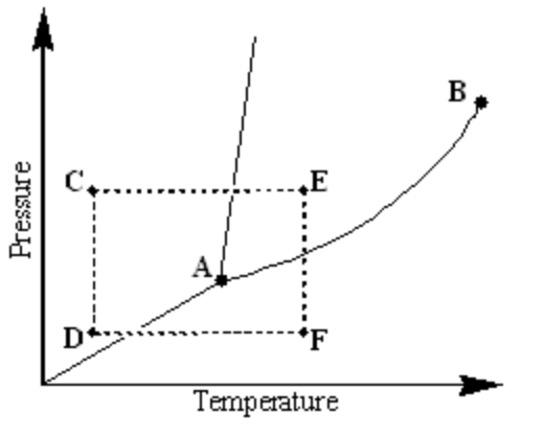Examine the following phase diagram and identify the phase change occurring as one goes from point D to point F.

- deposition caused by increasing the temperature

- sublimation caused by increasing the temperature

- condensation caused by increasing the temperature

- melting caused by increasing the temperature

- vaporization caused by increasing the temperature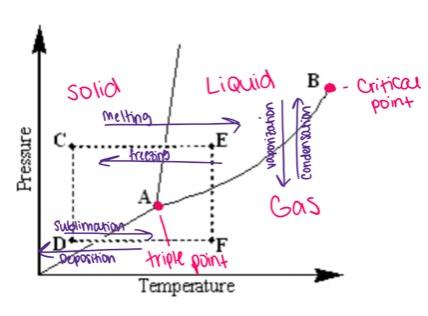sublimation caused by increasing the temperature

4

the band gap between the valence and conduction bands of semiconductors

- is large

- is non-existent

- decreases with increasing temperature

- is relatively small

- increases with increasing temperature

is relatively small

5

which of the following substances will have hydrogen bonds between the molecules?

- CH3SH

- CH3NH2

- CH3CH2Cl

- (CH3)2O

- HCl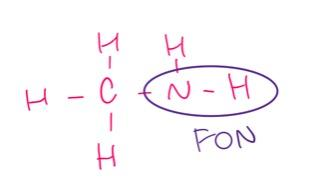CH3NH2

6

From the following list of aqueous solutions and water, select the one with the highest boiling point. (Note: Use the ideal values of the van't Hoff factor.)

- 0.37 M AlBr3

- 0.74 M SrBr2

- 0.95 M NaBr

- 0.57 M Na2S

- pure water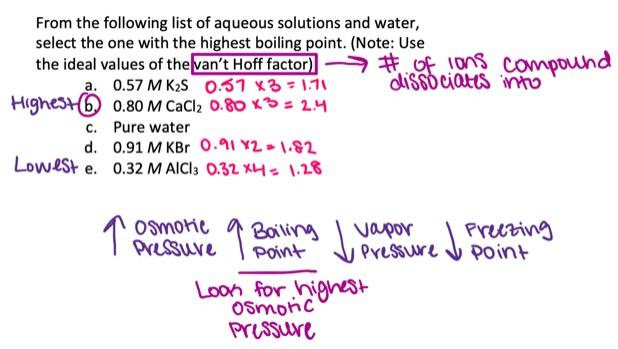0.74 M SrBr2

7

The boiling point of the molecular compound A is 55.2°C. How much energy is needed to heat 17.5 g of A from 12.5°C to 91.8°C?

Specific heat capacity of A(l )

3.52 J/(g⋅K)

Specific heat capacity of A(g )

2.11 J/(g⋅K)

Heat of vaporization of A

45.7 kJ/mol

Molar mass of A

30.4 g/mol

- 28.6 kJ

- 34.0 kJ

- 38.8 kJ

- 30.3 kJ

- 41.1 kJ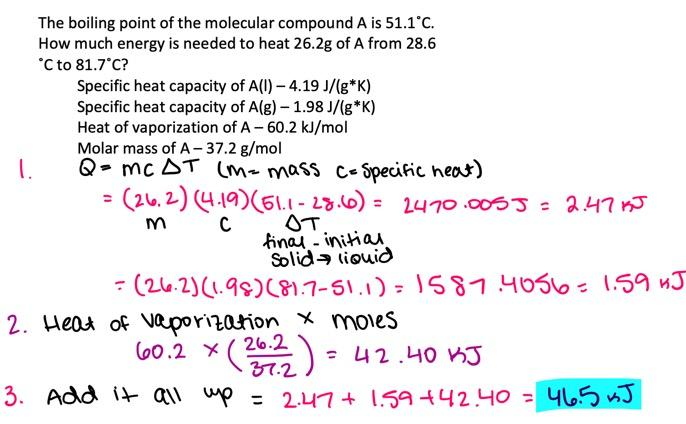30.3 kJ

8

The Henry's Law constant for carbon monoxide in water at 25°C is 9.71×10-4 mol/L·atm. How many moles of CO will dissolve in 1.00 L of water at this temperature if the partial pressure of CO is 382.1 torr?

- 0.00193 mol

- 0.000628 mol

- 0.000488 mol

- 0.000555 mol

- 0.371 mol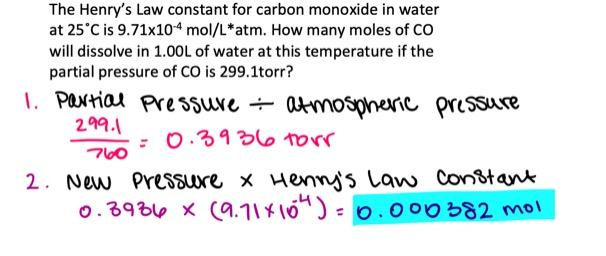0.000488 mol

9

A compound has vapor pressure of 29.5 torr at 41.7°C and 441.0 torr at 141.0°C. Calculate its heat of vaporization.

- 29.5 kJ/mol

- 25.8 kJ/mol

- 31.4 kJ/mol

- 36.5 kJ/mol

- 40.3 kJ/mol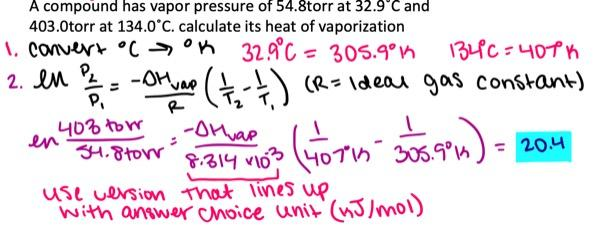29.5 kJ/mol

10

Which of the following statements is correct?

- Raoult's Law applies exactly to all solutions.-

- Raoult's Law relates the vapor pressure of the solvent above the solution to the molarity of the solution.

- Raoult's Law relates the vapor pressure of the solvent above the solution to its mole fraction in the solution.

- Raoult's Law applies only to non-ideal solutions.

- Raoult's Law works best when applied to concentrated solutions.

Raoult's Law relates the vapor pressure of the solvent above the solution to its mole fraction in the solution.

11

Determine the freezing point of a solution which contains 0.39 mol of sucrose in 93 g of water. (For water K f = 1.86°C/m and K b = 0.512°C/m)

- -2.1˚C

- -7.8˚C

- 0.0˚ C

- 2.1˚C

- 7.8˚C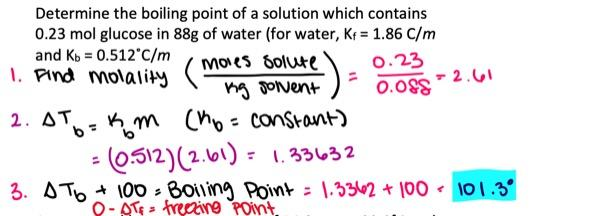-7.8˚C

12

Which of the following statements is correct?

- all of these statements are correct

- a solution becomes saturated when no more solute can be dissolved

- a solution becomes unsaturated when the solute precipitates in it

- none of these statements are correct

- supersaturated solutions are quite stable

a solution becomes saturated when no more solute can be dissolved

13

What is the coordination number for a metal that crystalizes in the face-centered cubic lattice?

- 4

- 2

- 8

- 6

- 12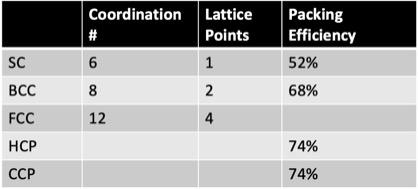12

14

Which of the following should have the highest boiling point?

- CH3CH3

- CH3SCH3

- CH3TeCH3

- CH3OCH3

- CH3SeCH3CH3TeCH3

15

Which of the following pairs of ions is arranged so that the ion with the smaller (i.e. less negative) heat of hydration is listed first?

- Al3+, Mg2+

- Ca2+, K+

- None of the above pairs are arranged in this way

- Sr2+, Ca2+

- S2–, Se2–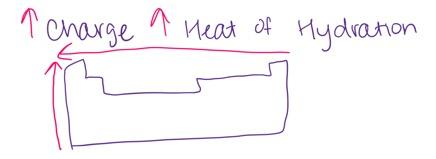Sr2+, Ca2+

16

Which of the following statements is correct with regard to the following two solutions? (Note: Use the ideal values of the van't Hoff factor.)

(I) 0.52 m RbCl and

(II) 0.21 m CaCl2

- Solution (I) has lower osmotic pressure

- The two solutions have the same vapor pressure

- Solution (I) has lower vapor pressure and lower osmotic pressure

- Solution (I) has higher osmotic pressure.

- Solution (II) has lower vapor pressure

Solution (I) has higher osmotic pressure.

17

A metal with a simple cubic lattice will have ________________ atom(s) per unit cell.

- 8

- 1

- 4

- 6

- 2

1

18

The heat needed to worm up a liquid depends on

- its vapor pressure

- its heat capacity

- the heat capacity of its vapors

- the value of the gas constant, R

- its normal boiling point

its heat capacity

19

An aqueous solution contains 3.77 g of the unknown compound X. The volume of the solution is 895.0 mL and its osmotic pressure is 2.85 atm at 25.0°C. Assuming that X is a nonvolatile nonelectrolyte, what is the molar mass of this compound?

- 36.2 g/mol

- 30.5 g/mol

- 27.9 g/mol

- 33.7 g/mol

- 50.4 g/mol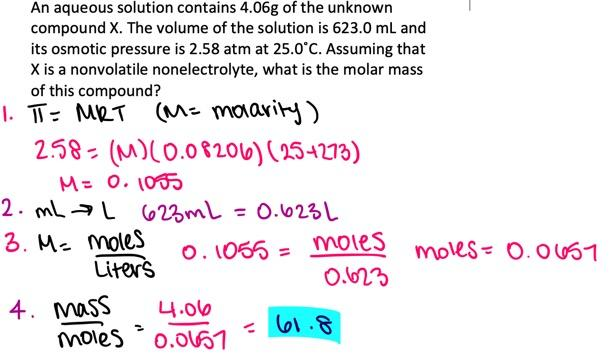36.2 g/mol

20

What is the molality of a solution prepared by dissolving 13.3 g of hexane, C6H14, in 71.0 g of benzene, C6H6?

- 2.17 m

- 1.57 m

- 2.57 m

- 2.05 m

- 2.74 m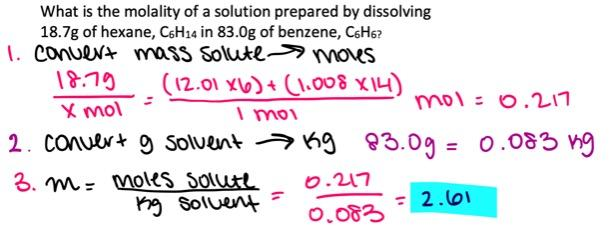2.17 m

21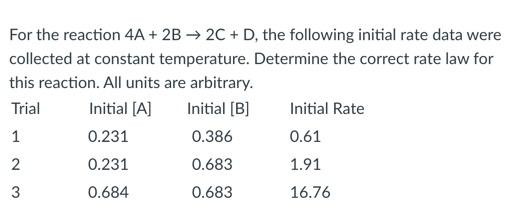- rate = k

- rate = k[A]2

- rate = k[B]2

- rate = k[A]2[B]2

- rate = k[A][B]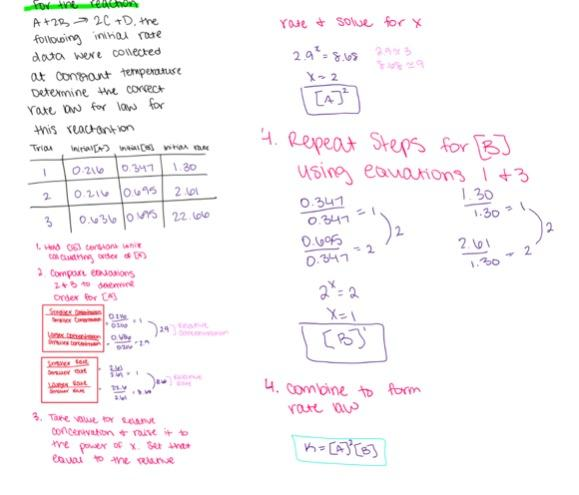rate = k[A]2[B]2

22

Which of the following statements about the reaction quotient, Q, and the equilibrium constant, K, is true?

- Q can not be affected by the total pressure.

- K is affected by the presence of a catalyst but Q is not.

- At equilibrium Q reaches a maximum.

- Q and K are basically the same thing.

- K is related to the rate constants of the forward and reverse reactions.

K is related to the rate constants of the forward and reverse reactions.

23

At 25oC, the equilibrium constant, for the reaction
3A(g) ↔ B(g) + C(g)
is K c = 670.0. What is the value of Kp? (R = 0.08206 L⋅atm/mol⋅K)

- 22.6

- 27.4

- 31.4

- 20.9

- 19.4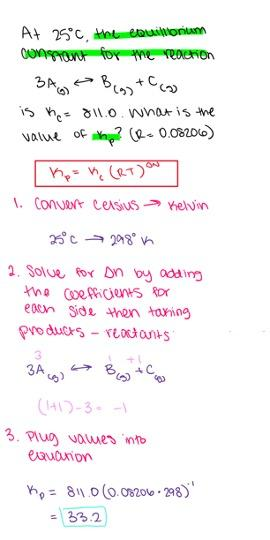27.4

24

The equilibrium constant, K c , for the reaction

2A(g) + B(g) ↔ 2C(g) + D(g)

is 6.94 at a certain temperature. What is the equilibrium constant for the following reaction at the same temperature?
C(g) + 1/2 D(g) ↔ A(g) + 1/2 B(g)

- 3.47

- 0.0208

- 0.380

- 0.443

- 1.86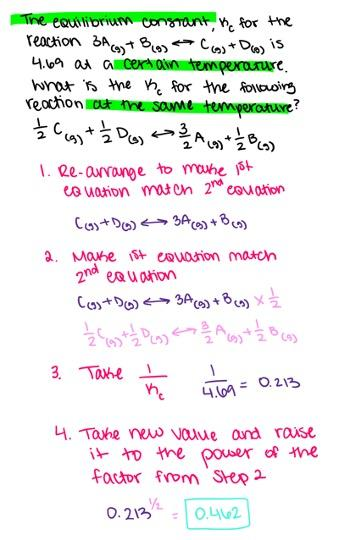0.380

25

Consider the following reaction
4A(g) + 4B(g) → 8C(g) + 5D(g)

If [A] is decreasing at the rate of 1.59 mol L-1s-1, at what rate is [D] changing?

- 1.77 mol L-1s-1

- 1.42 mol L-1s-1

- 1.99 mol L-1s-1

- 2.98 mol L-1s-1

- 1.14 mol L-1s-1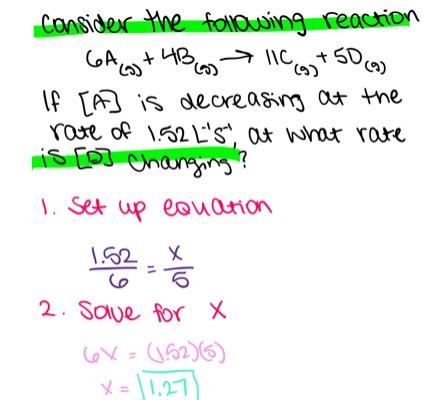1.99 mol L-1s-1

26

Consider the following reaction.
2A(g) + B(g) ↔ 4C(g) ΔHºrxn = –84 kJ/mol
Under which reaction conditions would you expect to have the greatest equilibrium yield of C(g)?

- low temperature, high pressure

- high temperature, low pressure

- high temperature, high pressure

- low temperature, low pressure

- none of the above, unless a catalyst is present

low temperature, low pressure

27

What is the mass-action expression, Kc , for the following chemical reaction?

PbO(s) + CO(g) ↔ Pb(l) + CO2(g)

- none of the above expressions is correct

- [CO2]/[CO]

- [Pb][CO2]/[PbO][CO]

- [Pb][CO2]/[CO]

- [CO]/[CO2]

[CO2]/[CO]

28

Compound A decomposes to B and C according to the following equation:
2A(g) ↔ B(g) + 2C(g)
At 100°C, 1.61 mol of A were placed in a 5.50 L container, and the reaction was allowed to proceed. After equilibrium was established, it was found that 66.6 % of A had decomposed. What is the value of K c for this reaction at 100°C?

- 0.363

- 0.388

- 0.281

- 0.454

- 0.498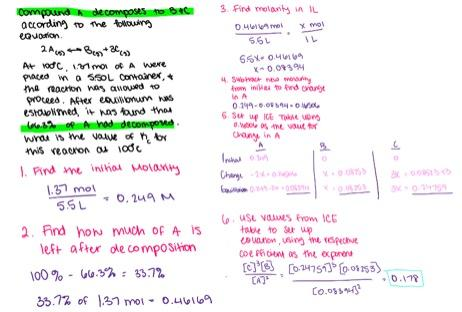0.388

29

The rate law for the reaction 2A → B + C is rate = k[A]2 with a rate constant k = 0.3765 L mol-1 s-1. If the initial concentration of A is 0.591 mol/L, calculate its concentration 1.69 seconds after the start of the reaction.

- 0.420 mol/L

- 0.462 mol/L

- 0.553 mol/L

- 0.593 mol/L

- 0.514 mol/L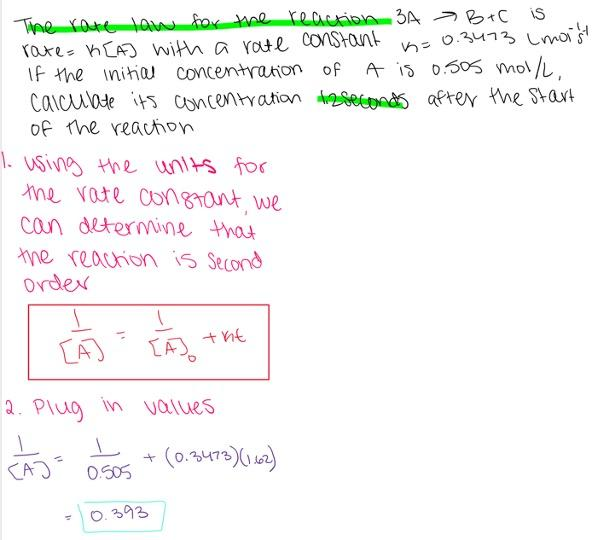0.420 mol/L

30

When solid magnesium hydroxide is added to water, part of it dissociates into magnesium and hydroxide ions.

Mg(OH)2(s) ↔ Mg2+(aq) + 2OH(aq)

If the above reaction is at equilibrium at a certain temperature, what would be the effect of adding a small amount of magnesium ions to the solution?

- The solution will become supersaturated.

- The forward reaction will proceed to establish equilibrium.

- The reverse reaction will proceed to establish equilibrium.

- None of the above conclusions is justified without additional information.

- No effect.

The reverse reaction will proceed to establish equilibrium.

31

The the units of the rate constant for the reaction A + B → C + D are L2 mol-2 s-1. What is the overall order of the reaction?

- first

- none of the above

- zero

- second

- third

third

32

If the activation energy of a certain chemical reaction is 31.3 kJ/mol, by what factor will the rate of this reaction increase when the temperature is increased from 32 oC to 64 oC? (R = 8.314×10-3 kJ/mol⋅K)

- 3.48

- 2.35

- 3.23

- 3.81

- 2.49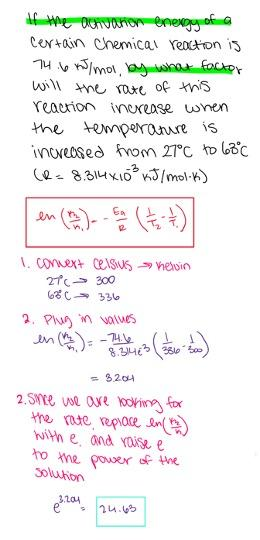3.23

33

When the reaction A → B + C is studied, a plot of 1/[A] versus time gives a straight line with a positive slope. What is the the order of the reaction?

- first

- third

- second

- zero

second

34

At a certain temperature, the equilibrium constant for the reaction
2NaHCO3(s) ↔ Na2CO3(s) + H2O(g) + CO2(g)
is K p = 0.320. If a sufficient quantity of NaHCO3(s) is placed in a closed container at this temperature, what would be the partial pressure of water vapor after equilibrium is established?

- 0.766 atm

- 0.566 atm

- 0.473 atm

- 0.531 atm

- 0.715 atm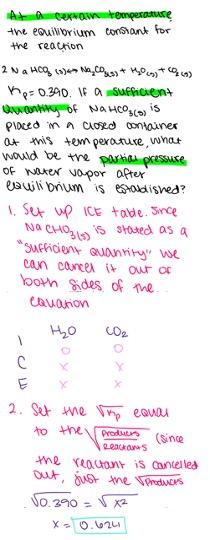0.566 atm

35

At 25oC, the equilibrium constant for the reaction
3A(g) ↔ B(g) + C(g)
is Kp = 0.0598. If the three gases are mixed in a rigid container having partial pressures of 2.26, 1.19 and 0.266 atm for A, B and C, respectively, is the system at equilibrium?

- the reverse reaction must proceed to establish equilibrium

- the forward reaction must proceed to establish equilibrium

- the volume of the container must be known to answer the question

- the concentrations of all substances must be known to answer the question

- the system is at equilibrium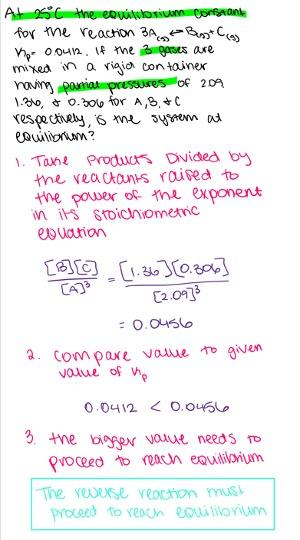the forward reaction must proceed to establish equilibrium

36

Which of the following statements is true?

- A catalyst accelerates a reaction by changing the reaction pathway.

- A catalyst accelerates a reaction by supplying energy to the reactant molecules.

- A catalyst accelerates a reaction by increasing the number of molecular collisions.

- A catalyst accelerates a reaction by increasing its activation energy.

- A catalyst accelerates a reaction and is consumed in it.

A catalyst accelerates a reaction by changing the reaction pathway.

37

A particular radioactive isotope undergoes a first order decay with a half-life of 25.1 years. How long would it take for the concentration of this isotope to drop to 53.6 % of its original value?

- 30.9 years

- 29.3 years

- 24.8 years

- 26.3 years

- 22.6 years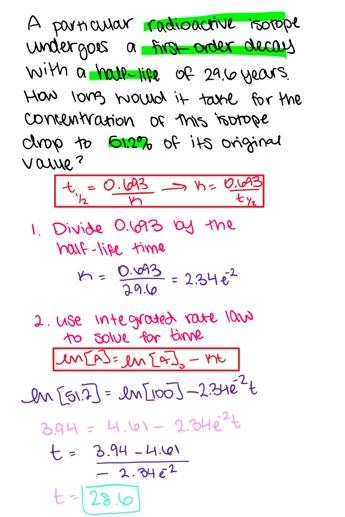22.6 years

38

The experimental rate law for the reaction 2A + 2B → D + E is Rate = k[A]2 [B]2. Which of the following statements is correct for the mechanism suggested below?
2A + B → 3C + D (slow)
3C + B → E (fast)

- none of the above is correct

- the mechanism should only have a single step

- the mechanism is not consistent with the rate law

- The mechanism is consistent with the rate law but it is not physically reasonable

- The mechanism is consistent with the rate law and it is physically reasonable

the mechanism is not consistent with the rate law

39

The rate law for the reaction A → B is rate = k[A] with a rate constant of 2.70 s-1. If the initial concentration of A is 0.632 mol/L, what is the half-life of the reaction?

- 0.326 s

- 0.092 s

- 0.586 s

- 0.117 s

- 0.257 s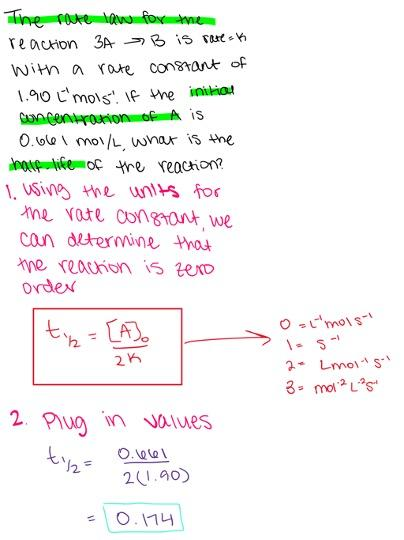0.257 s

40

At a certain temperature, the equilibrium constant for the reaction
CO2(g) + H2(g) ↔ CO(g) + H2O(g)
is K c = 3.98. If 2.00 mol of CO2 and 2.00 mol of H2 are placed in a 2.00 L vessel and equilibrium is established, what will be the concentration of water?

- 0.922 M

- 0.792 M

- 0.531 M

- 0.703 M

- 0.666 M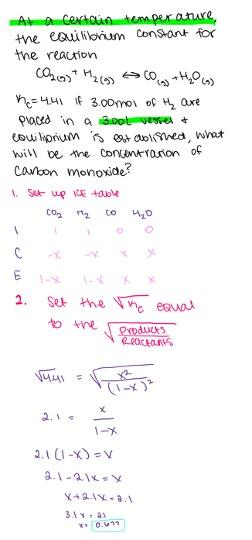0.666 M

41

Which of the following acids and its conjugate base should be used to prepare an efficient buffer with a pH = 4.0?
(1) HClO2, K a = 1.1×10-2
(2) CH3COOH, K a = 1.8×10-5
(3) HClO, K a = 2.9×10-8

- 1

- 3

- 2

- both 2 and 3 would be fine

- both 1 and 2 would be fine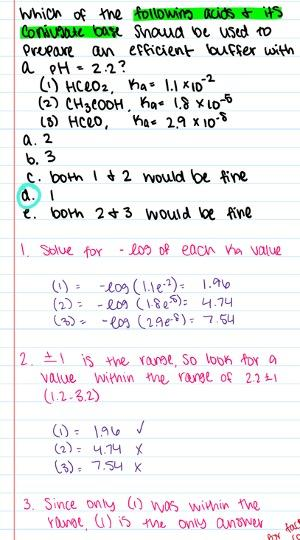2

42

What is the pH of a 1.9×10–5 M Ca(OH)2 solution?

- 8.96

- 9.28

- 10.77

- 9.58

- 11.36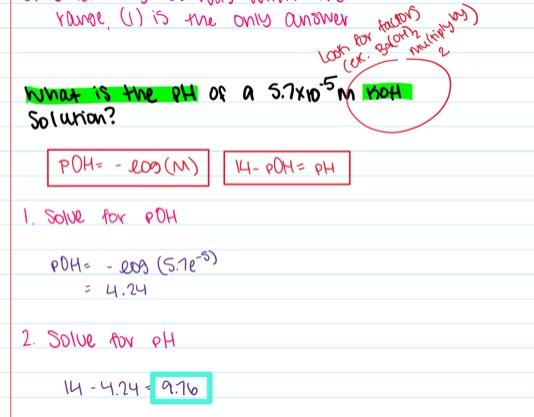9.58

43

What is the pK a of the alkylammonium ion R3NH+? (K b = 4.6×10–5 for R3N)

- 9.66

- 3.68

- 5.55

- 4.34

- 9.07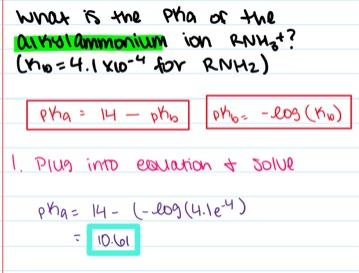9.66

44

The organic acid RCOOH has a pKa = 4.14. What is the pH of a 0.40 M aqueous solution of this acid?

- 3.15

- 2.27

- 2.09

- 2.88

- 1.96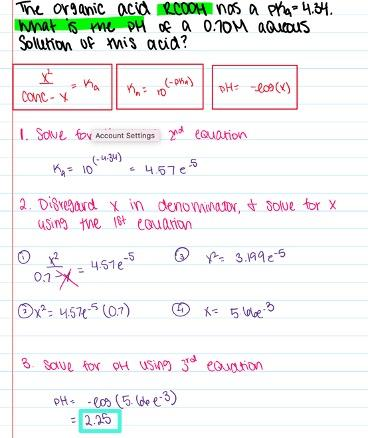2.27

45

Sodium nitrite, NaNO2, is best known as a food additive. What is the pH of a 0.350 M NaNO2 solution in water? (K a of HNO2 is equal to 7.1×10-4)

- 7.00

- 5.65

- 8.35

- 5.15

- 8.61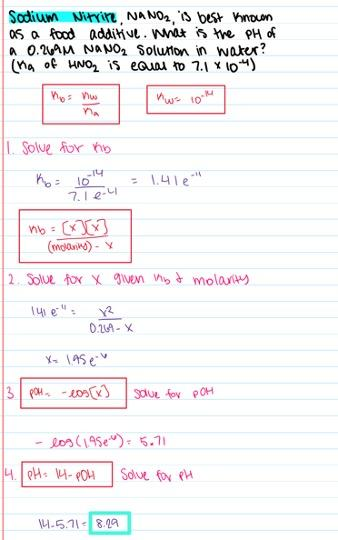8.35

46

Calculate the molar solubility of barium sulfate, BaSO4, in 0.34 M BaCl2 solution. (K sp = 1.1×10-10 for BaSO4)

- 1.9e-10 M

- 6.2e-11 M

- 2.4e-9 M

- 3.2e-10 M

- 9.1e-11 M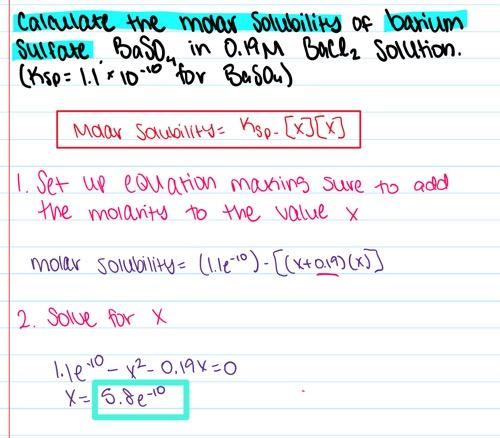3.2e-10 M

47

Select the pair of substances in which an acid is listed followed by its conjugate base.

- NH4 +, NH2

- H2CO3, CO3 2-

- H+, HF

- H3PO4, HPO4 2–

- HCO3 , CO3 2-

HCO3 , CO3 2-

48

Calculate the molar solubility of Fe(OH)2 in pure water. (K sp = 4.1×10–15)

- 1.6e–5 M

- 6.4e–8 M

- 1.1e–4 M

- 5.2e–4 M

- 1.0e–5 M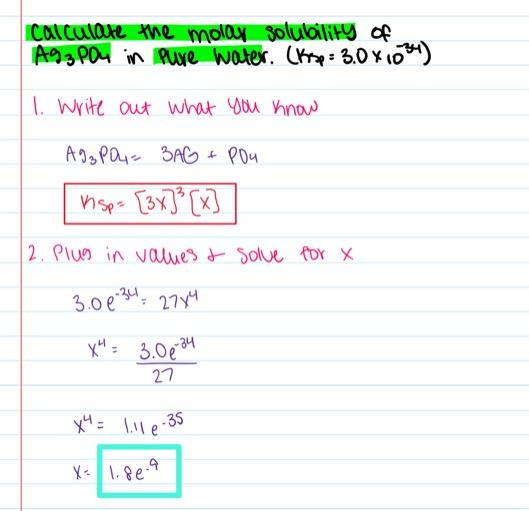1.0e–5 M

49

A solution is prepared by adding 0.034 mol of NaCl to 1.00 L of 0.0114 M Pb(NO3)2. Which of the following statements is correct? (K sp = 1.7×10-5 for PbCl2)

- The solution is unsaturated and no precipitate forms.

- Both sodium nitrate and lead(II) chloride precipitate.

- Lead(II) chloride precipitates until the solution is saturated.

- Sodium nitrate precipitates until the solution is saturated.

- The data is not sufficient to predict precipitation.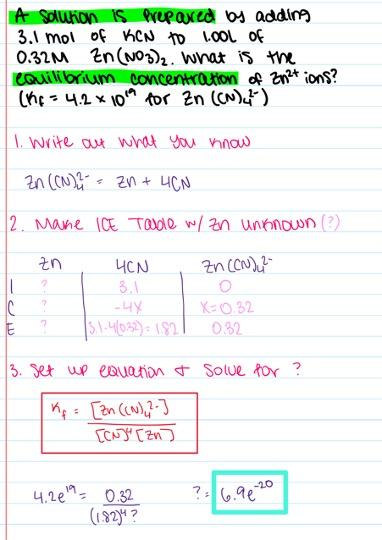The solution is unsaturated and no precipitate forms.

50

An aqueous solution of NH4Cl would be

- either acidic or basic depending on the amount of salt dissolved.

- neutral

- either acidic or basic depending on the actual values of K a and K b which must be known.

- acidic

- basic

acidic

51

The species NH3 is classified as a(n)

- none of the above

- Lewis acid

- Arrhenius base

- Arrhenius acid

- Lewis base

Lewis base

52

A 0.41 M solution of a given weak acid, HA, has a pH of 4.81. What is the pKa of the acid?

- 12.66

- 8.67

- 7.97

- 11.55

- 9.23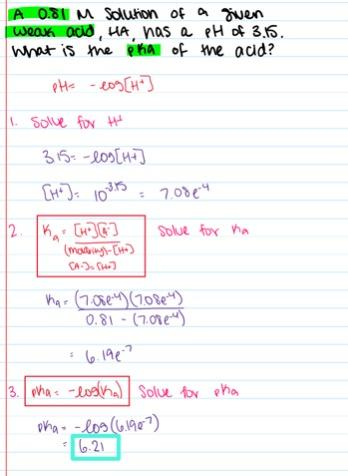9.23

53

What is the pH of a buffer that consists of 0.32 M HClO and 0.67 M NaClO? (K a(HClO) = 2.9×10–8) - 7.17

- 10.98

- 10.13

- 9.16

- 7.86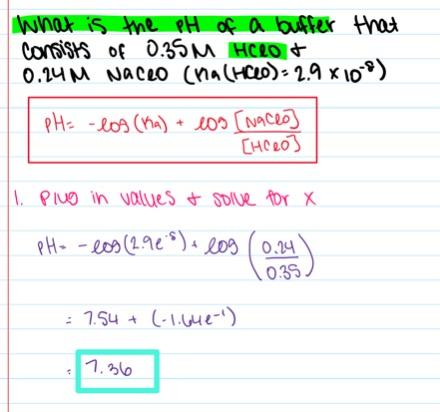7.86

54

The acid ionization constant of H2SO3 is K a = 1.4×10–2 and that of HClO is K a = 2.9×10–8. Which statement about the equilibrium below is correct?
H2SO3(aq) + ClO(aq) ↔ HSO3 (aq) + HClO(aq)

- The initial concentrations of the reactants must be known before any prediction can be made.

- The reactants will be favored at equilibrium.

- Neither reactants nor products will be favored because all of the species are weak acids or bases.

- The products will be favored at equilibrium.

- Neither reactants nor products will be favored because the reaction is impossible.

The products will be favored at equilibrium.

55

When a strong base is titrated with a strong acid, the pH at the equivalence point is

- strongly acidic

- neutral

- slightly acidic

- slightly basic

- strongly basic

neutral

56

Which of the following arrangements has the strongest acid listed first and the weakest acid listed last?

- H3N > HF > H2O

- HF > H2O > H3N

- H2O > HF > H3N

- HF > H3N > H2O

- H2O > H3N > HF

HF > H2O > H3N

57

A particular acid-base indicator has an acid ionization constant, K In = 6.4×10–9. What would be the approximate pH range over which it changes color?

- 7.2-92

- 8.2-10.2

- 5.2-7.2

- 4.2-6.2

- 6.2-8.2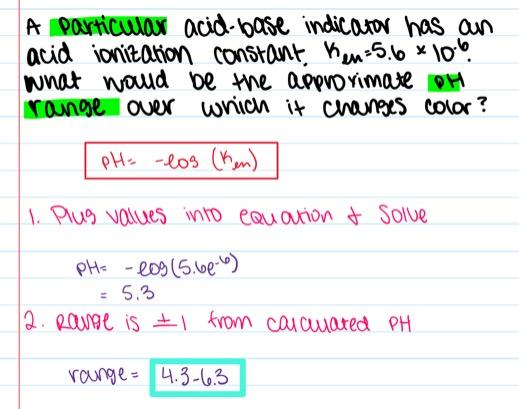7.2-9.2

58

A solution is prepared by adding 2.6 mol of KCN to 1.00 L of 0.30 M Zn(NO3)2. What is the equilibrium concentration of Zn2+ ions? (K f = 4.2×1019 for Zn(CN)4 2-)

- 2.0e-22 M

- 1.4e–20 M

- 1.9e–21 M

- 6.2e–21 M

- 1.1e–20 M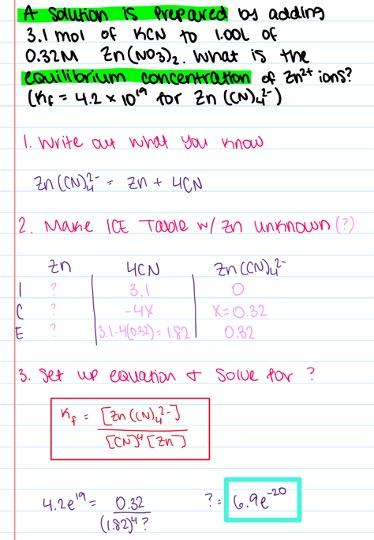1.9e–21 M

59

Calculate the pH during the titration of 34.0 mL 0.103 M formic acid (pKa = 3.74) with 0.110 M NaOH after the addition of 17.0 mL of the titrant.

- 3.52

- 3.28

- 3.80

- 4.21

- 3.94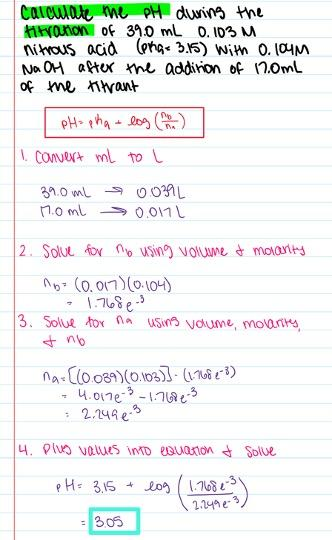3.80

60

Which, if any, of the following aqueous mixtures would be buffer systems?

(1) HNO2 , KH2PO4

(2) H2SO3 , NaHSO3

- 1 is a buffer but 2 is not

- neither 1 nor 2 are buffers

- none of above

- 2 is a buffer but 1 is not

- both 1 and 2 are buffers

2 is a buffer but 1 is not

61

How long would it take to deposit 8.43 g of copper (molar mass 63.55 g/mol) at the cathode of an electrolytic cell, if a current of 14.7 A is passed through an aqueous solution of CuSO4?

- 38.7 min

- 44.8 min

- 29.0 min

- 24.0 min

- 25.5 min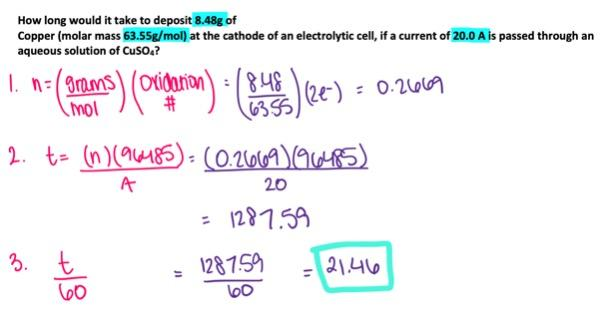29.0 min

62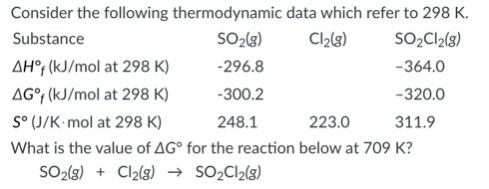- 19.8 kJ

- 45.7 kJ

- -67.2 kJ

- -45.7 kJ

- 19.8 kJ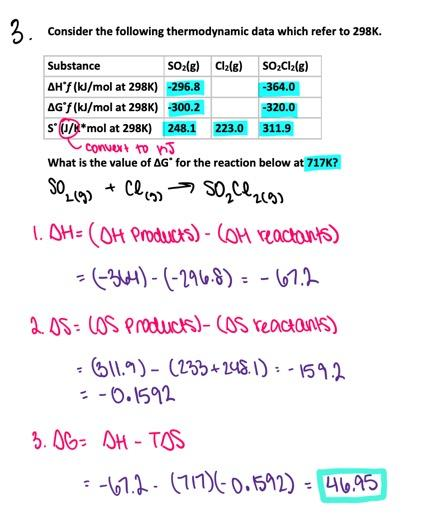45.7 kJ

63

Predict the sign of ΔSo for the following reaction.

C2H5OH (l) + 3O2(g) → 2CO2 (g) + 3H2O (l)

- ∆S˚ > 0

- ∆S˚ ≈ 0

- ΔSo < 0

- ΔSo could be positive or negative depending on the sign of ΔH°.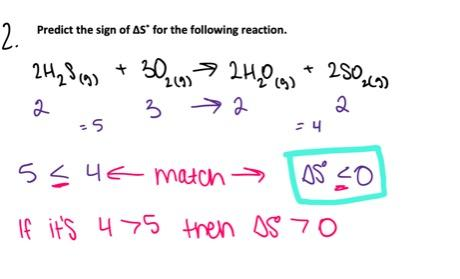ΔSo < 0

64

Consider the following redox equation
MnO4 (aq) + ClO3 (aq) → Mn2+(aq) + ClO4 (aq) (acidic solution)
When the equation is balanced with smallest whole number coefficients, what is the coefficient of the permanganate ion?

- 3

- 4

- 1

- 2

- 5

2

65

If Eo cell = 0.30 V for the reaction below at 25oC, what is the value of the equilibrium constant at this temperature.

Sn2+ (aq) + Fe(s) ↔ Fe2+ (aq) + Sn(s)

- 2.2e0

- 7.3e-11

- 1.0e0

- 1.4e10

- 1.2e5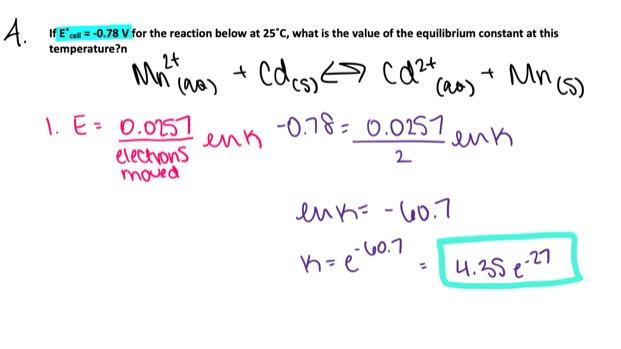1.4e10

66

Use the table of standard reduction potentials attached to your test to calculate Eo cell for the following reaction and determine if it is spontaneous at standard conditions.

Ca2+ (aq) + Cd(s) → Cd2+ (aq) + Ca(s)

- Eo cell = –2.47 V, spontaneous

- Eo cell = 2.47 V, spontaneous

- Eo cell = –3.27 V, non-spontaneous

- Eo cell = 2.47 V, non-spontaneous

- Eo cell = –2.47 V, non-spontaneous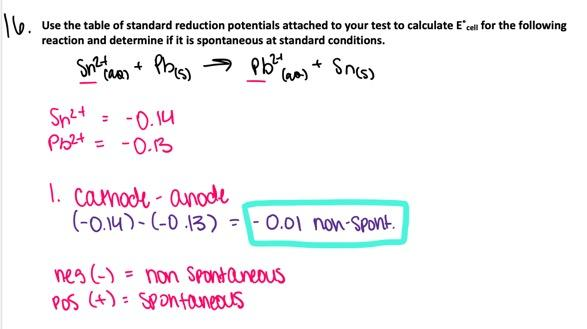Eo cell = –2.47 V, non-spontaneous

67

Which, if any, of the following two elements can be isolated by electrolysis of the the aqueous salt shown?
(1) Ni from NiSO4 (aq)
(2) Br2 from NaBr(aq)

- Neither (1) nor (2) can be isolated.

- Both (1) and (2) can be isolated.

- (1) can not be isolated but (2) can be isolated

- (1) can be isolated but (2) can not be isolated.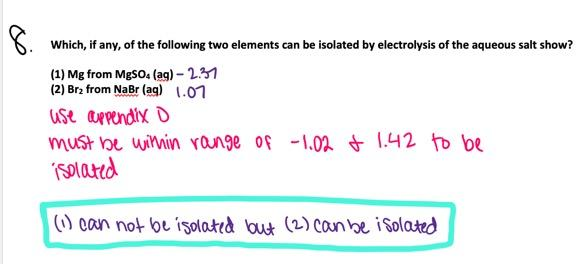Both (1) and (2) can be isolated.

68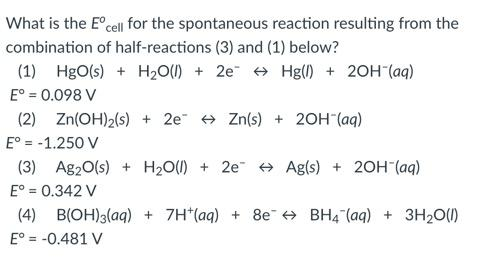- 0.244 V

- -0.244 V

- 0.220 V

- -0.440 V

- 0.440 V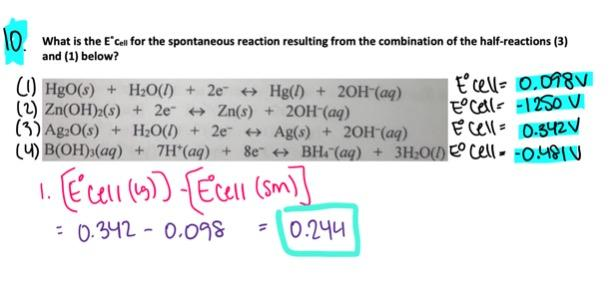0.244 V

69

For a given chemical reaction, ΔΗ > 0 and ΔS < 0. Therefore,

- The reaction is spontaneous only at high temperatures.

- The reaction is non-spontaneous at all temperatures.

- The reaction is spontaneous only at low temperatures.

- The reaction is spontaneous at all temperatures.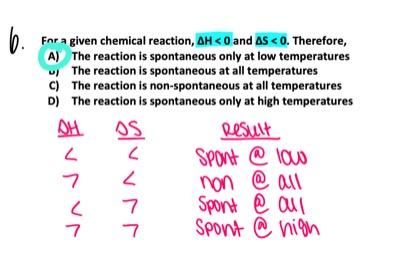The reaction is non-spontaneous at all temperatures.

70

For the reaction below, ΔGo = 24.0 kJ/mol at 989 K. If at 989 K, an excess of the solid A(s) is added to the gases B(g) and C(g) both having partial pressures of 0.76 atm, which statement is true?
A(s) → 2B(g) + 2C(g)

- The reverse reaction will proceed spontaneously.

- The reaction will be at equilibrium.

- The reaction quotient will become negative.

- The equilibrium constant will increase.

- The forward reaction will proceed spontaneously.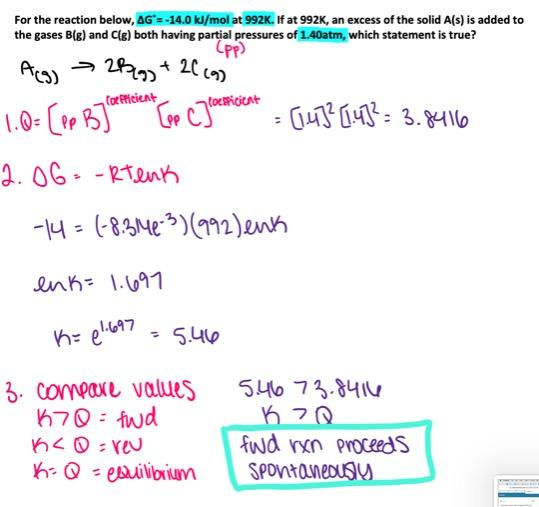The reverse reaction will proceed spontaneously.

71

Which one of the following statements about electrochemical cells is correct?

- The free energy change is negative for an electrolytic cell.

- The cathode is labeled as negative (-) in a voltaic cell but positive (+) in an electrolytic cell.

- Reduction occurs at the cathode of both galvanic and electrolytic cells.

- In the salt bridge cations travel toward the anode and anions toward the cathode.

- None of the above statements is correct.

Reduction occurs at the cathode of both galvanic and electrolytic cells.

72

A galvanic cell consists of an Au/Au3+ electrode (Eo = +1.50 V) and a Ag/Ag+ electrode (Eo = +0.80 V). Calculate the cell potential, E cell, at 25oC if [Au3+] = 6.9×10–7 M and [Ag+] = 3.6×10–4 M.

- 0.73 V

- 0.81 V

- 0.70 V

- 0.78 V

- 0.89 V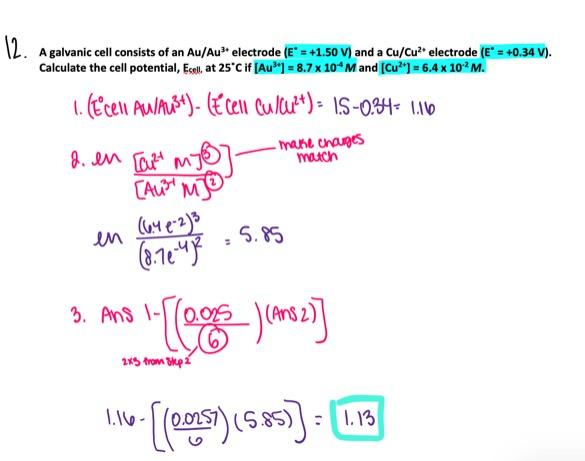0.78 V

73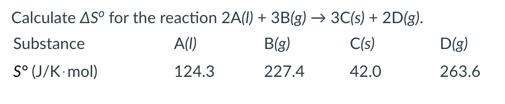- 657.3 J/K

- 46.1 J/K

- -277.6 J/K

- 277.6 J/K

- -46.1 J/K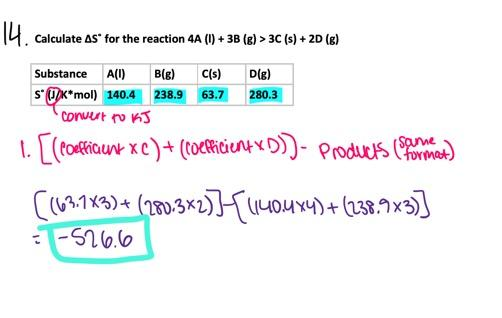- 277.6 J/K

74

Which of the following relationships best describes ΔSo for the following reaction.

HCl (g) → H+(aq) + Cl- (aq)

- ΔSo ≈ 0

- ΔSo > 0

- ΔSo = TΔH°

- ΔSo < 0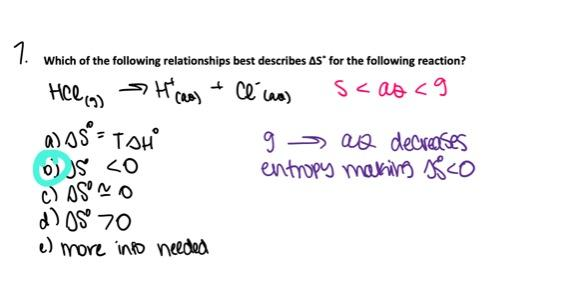ΔSo < 0

75

Examine the table of standard reduction potentials attached to your test and determine which of the following species is the strongest oxidizing agent?

- Sn2+ (aq)

- Sn(s)

- Sn4+ (aq)

- Au3+ (aq)

- NO3 (aq)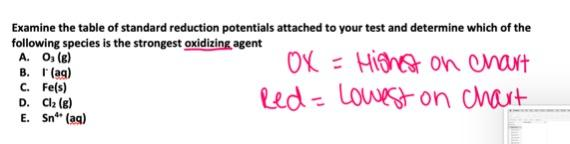Au3+ (aq)

76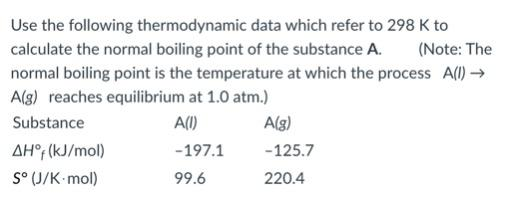- 521.4 K

- 718.9 K

- 454.6 K

- 591.1 K

- 269.9 K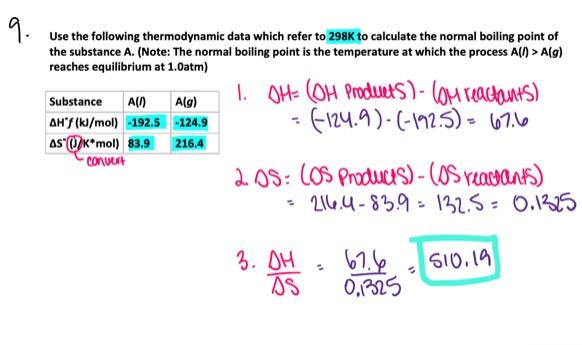591.1 K

77

Which of the following should have the greatest molar entropy at 298 K?

- Cl2 (aq)

- F2 (g)

- Cl2 (g)

- all should have the same molar entropy since the temperature is constant

- F2 (aq)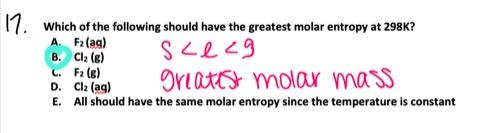Cl2 (g)

78

For the reaction below, ΔHr o = 178.5 kJ/mol and ΔSr o = 159.0 J/mol⋅K at 298 K. What is the equilibrium constant of this reaction at 572.0 K?
CaCO3 (s) ↔ CaO(s) + CO2 (g)

- 1.9e-8

- 7.0e-9

- 1.0e-8

- 8.4e-9

- 6.2e-9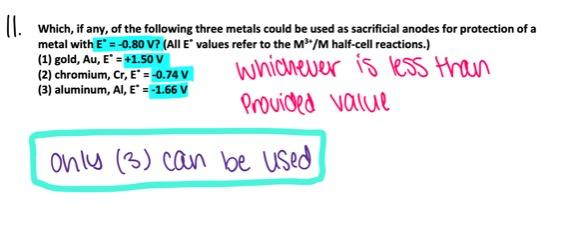1.0e-8

79

A galvanic cell has the following notation:

Sn(s) | Sn2+(aq) || Fe3+(aq), Fe2+(aq) | Pt(s)

Which of the following equations correctly represents the overall cell reaction?

- Sn(s) + Fe2+(aq) → Sn2+(aq) + Fe3+(aq)

- Sn2+(aq) + Fe2+(aq) → Sn(s) + Fe3+(aq)

- Sn(s) + 2Fe3+(aq) → Sn2+(aq) + 2Fe2+(aq)

- Sn2+(aq) + 2Fe3+(aq) → Sn(s) + 2Fe2+(aq)

- Sn(s) + Fe3+(aq) → Sn2+(aq) + Fe2+(aq)

Sn(s) + 2Fe3+(aq) → Sn2+(aq) + 2Fe2+(aq)

80

Which, if any, of the following three metals could be used as sacrificial anodes for protection of a metal with E° = 0.93 V? (All E° values refer to the M3+/M half-cell reactions.)
(1) gold, Au, E° = +1.50 V
(2) chromium, Cr, E° = -0.74 V
(3) aluminum, Al, E° = -1.66 V

- none of them could be used

- only (3) could be used

- all three of them could be used

- only (1) and (2) can be used

- only (2) and (3) could be usedonly (2) and (3) could be used